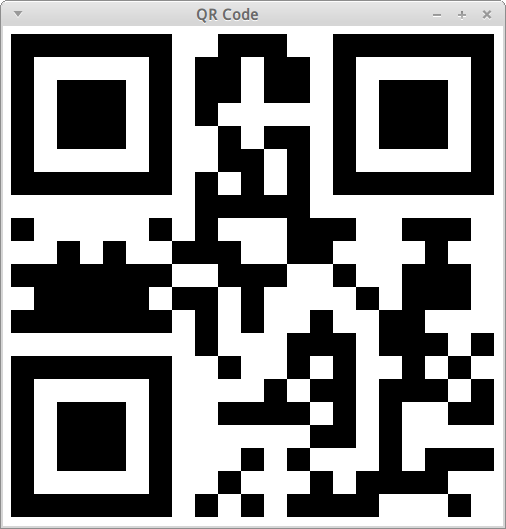MagicQRCode函式庫的使用方法十分簡單，只要兩行就可以產生出QR Code：

```QRCodeEncoder qr = new QRCodeEncoder("QR Code的內容");
boolean[][] qrData = qr.encode();```

```QRCodeEncoder qr = new QRCodeEncoder("QR Code的內容");
qr.setErrorCorrect(QRCodeEncoder.ErrorCorrect.MAX);
boolean[][] qrData = qr.encode();```

qrData是一個二維的boolean陣列，陣列的大小會根據編碼的文字長度和容錯率而有所變動，但這個陣列一定是個方形陣列(長度相同)。如果要將qrData轉成我們平常看到由黑白兩色組成的圖片，可以參考以下程式碼，將其會製成圖形：

##### JavaFX繪製QR Code
```public static void drawQRCode(final Canvas canvas, final boolean[][] qrData) {
final int width = (int)canvas.getWidth();
final int height = (int)canvas.getHeight();
final GraphicsContext gc = canvas.getGraphicsContext2D();

//畫背景(全白)
gc.setFill(Color.WHITE);
gc.fillRect(0, 0, width, height);

final int imageSize = Math.min(width, height);
final int length = qrData.length;
final int size = imageSize / length;
final int actualImageSize = size * length;
final int offsetImageX = (width - actualImageSize) / 2;
final int offsetImageY = (height - actualImageSize) / 2;

//畫資料(true為黑色)
gc.setFill(Color.BLACK);
for (int i = 0; i < length; i++) {
for (int j = 0; j < length; j++) {
if (qrData[i][j]) {
final int x = i * size + offsetImageX;
final int y = j * size + offsetImageY;
gc.fillRect(x, y, size, size);
}
}
}
}```##### Android SDK繪製QR Code
```public static void drawQRCode(final Canvas canvas, final boolean[][] qrData) {
final Paint paint = new Paint();
final int width = canvas.getWidth();
final int height = canvas.getHeight();

//畫背景(全白)
paint.setColor(Color.WHITE);
canvas.drawRect(new Rect(0, 0, width, height), paint);

final int imageSize = Math.min(width, height);
final int length = qrData.length;
final int size = imageSize / length;
final int actualImageSize = size * length;
final int offsetImageX = (width - actualImageSize) / 2;
final int offsetImageY = (height - actualImageSize) / 2;

//畫資料(true為黑色)
paint.setColor(Color.BLACK);
for (int i = 0; i < length; i++) {
for (int j = 0; j < length; j++) {
if (qrData[i][j]) {
final int x = i * size + offsetImageX;
final int y = j * size + offsetImageY;
canvas.drawRect(new Rect(x, y, x + size, y + size), paint);
}
}
}
}```